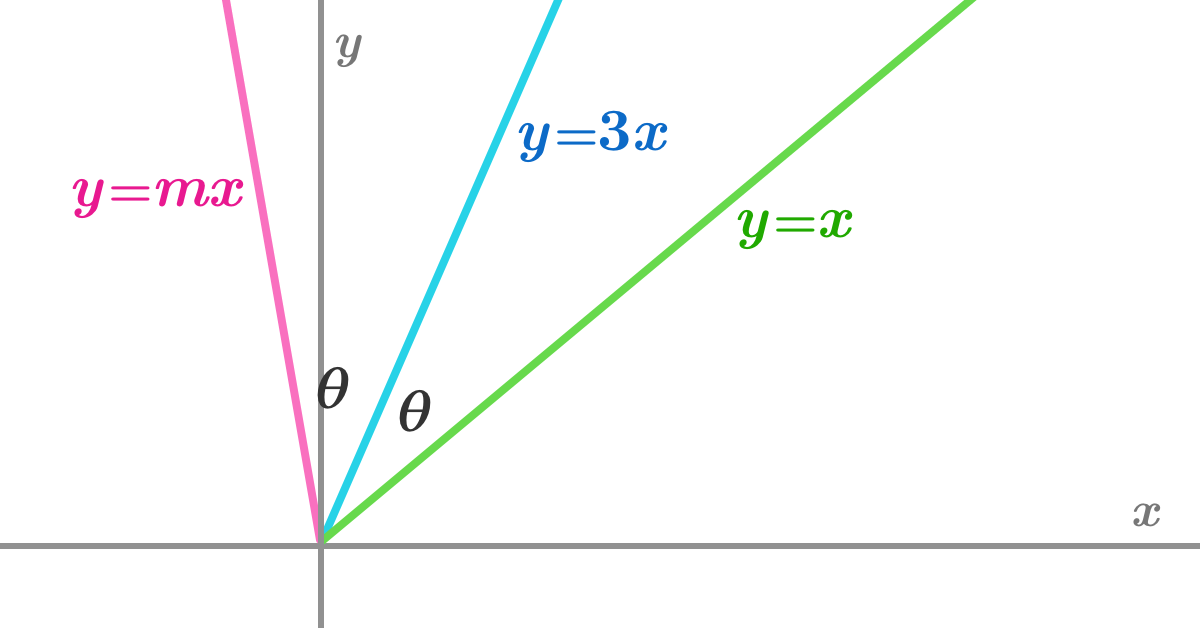# Tangents And GradientsIf $y=3x$ is the angle bisector of $y=x$ and $y=mx$, then find $m.$

×